## Tuesday, October 27, 2015

### Simulating a species tree from a genus tree in which the genera arise (stochastically) under a Yule process

Today, Karla Shikev asked the following question:

I need to add species go a genus-level phylogeny, such that the generated nodes are consistent with a Yule process. I tried phytools' add.species.to.genus function, but the generated branch lengths tend to be too recent.

`add.species.to.genus` adds tips randomly - not under some model. However, if we have a purely genus-level tree (that is, a tree in which only genera are represented) and we want to produce random species trees, in which each subtree arose by a Yule process - then this is not too hard either.

Here is a quick demo:

``````library(phytools)
## first let's simulate our genus tree:
genus.tree<-pbtree(n=7,tip.label=c("Abc","Def","Ghi","Jkl","Mno","Qrs","Tuv"))
## here it is:
plotTree(genus.tree,ftype="i")
``````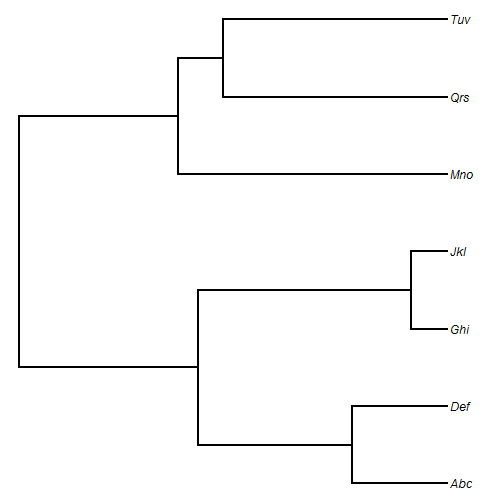Next, let's simulate some random species within each genus to attach to our tree. Here I simulate 1-5 species per genus; however, note that if there is only one species in a genus, then the tip of our genus tree will simply be renamed.

``````tips<-c()
for(i in 1:Ntip(genus.tree)){
n.genus<-sample(1:5,1)
for(j in 1:n.genus) tips<-c(tips,paste(genus.tree\$tip.label[i],paste(sample(letters,6),collapse=""),sep="_"))
}
## these are the tips we want for our species tree:
tips
``````
``````##   "Abc_svhmwl" "Abc_xhjdvy" "Def_ykhsrx" "Ghi_dxuhtl" "Ghi_avzyks"
##   "Ghi_buyawg" "Jkl_nbgmsk" "Jkl_ryialh" "Jkl_nwuqhe" "Jkl_ufmixj"
##  "Jkl_bclftw" "Mno_sonmkr" "Mno_kpydtl" "Mno_ujalsy" "Mno_cyeojk"
##  "Mno_sohent" "Qrs_xlbphe" "Qrs_nvsziu" "Qrs_iuwkjm" "Qrs_tscqbr"
##  "Qrs_azcfnl" "Tuv_tidfwy"
``````

OK, now let's imagine how we want to create our species tree in which each genus arose via a Yule process. We can simply take the genus tree and glue on a Yule tree somewhere along the terminal edge leading to each tip in the genus tree! Here is an example function in which this is done at a random position along that edge - but this needn't be the case, and could be modified easily:

``````genus.to.pbSpeciesTree<-function(tree,tips){
N<-Ntip(tree)
genera<-tree\$tip.label
for(i in 1:N){
jj<-grep(paste(genera[i],"_",sep=""),tips)
nn<-which(tree\$tip.label==genera[i])
if(length(jj)>1){
h<-runif(n=1)*tree\$edge.length[which(tree\$edge[,2]==nn)]
tree\$edge.length[which(tree\$edge[,2]==nn)]<-
tree\$edge.length[which(tree\$edge[,2]==nn)]-h
sub.tree<-pbtree(n=length(jj),scale=h,tip.label=tips[jj])
tree<-bind.tree(tree,sub.tree,where=nn)
} else tree\$tip.label[nn]<-tips[jj]
}
tree
}
species.tree<-genus.to.pbSpeciesTree(genus.tree,tips)
plotTree(species.tree,ftype="i")
``````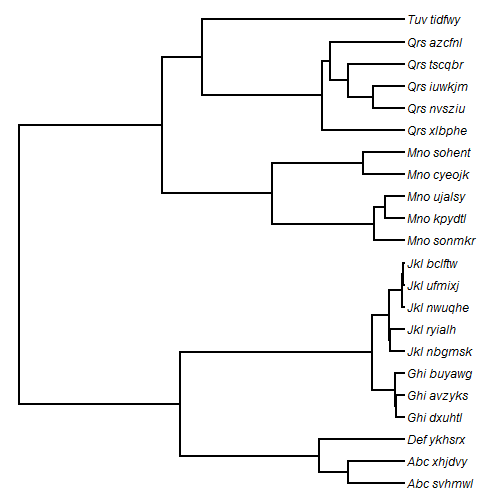As an alternative to rescaling, we could have simulated conditioned on time & a birth rate; however I'm not sure it would make much difference, and it would require that some birthrate be supplied.

That's it!

## Sunday, October 25, 2015

### New features in phytools function plotTree.wBars

On the suggestion of Frederico Faleiro I just added two new features to the phytools plotting function `plotTree.wBars`.

First, the user can now set the bar colors. This can be a single color, or different colors for different bars. Second, the user can now set or turn off bar borders.

Here's a quick demo:

``````library(phytools)
tree<-pbtree(n=60,scale=1)
x<-fastBM(tree)
## basic method:
plotTree.wBars(tree,x,scale=0.5)
``````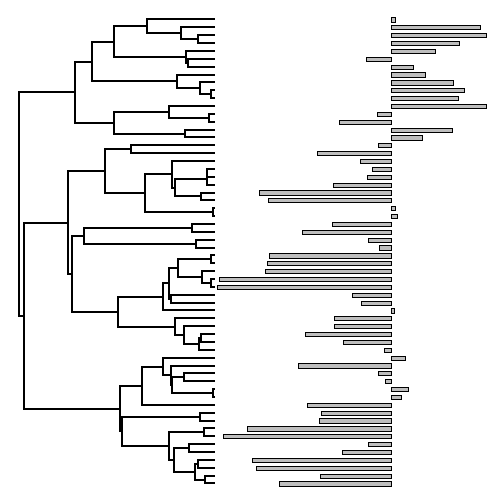Now, greyscale by value:

``````col<-grey((x-min(x))/(max(x)-min(x)))
col<-setNames(col,names(x))
col[1:10] ## e.g.
``````
``````##       t44       t45       t31       t32       t23       t24       t42
## "#3B3B3B" "#626262" "#252525" "#212121" "#767676" "#8F8F8F" "#060606"
##       t43       t34       t35
## "#1D1D1D" "#606060" "#626262"
``````
``````plotTree.wBars(tree,x,col=col,scale=0.5,border="grey")
``````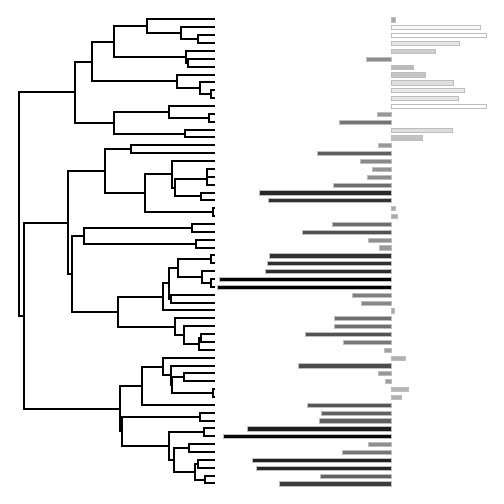Rainbow by value (a little trickier):

``````## just one way to do this:
obj<-contMap(tree,x,plot=FALSE)
foo<-function(i,obj){
ind<-which(obj\$tree\$edge[,2]==i)
n<-names(obj\$tree\$maps[[ind]])[length(obj\$tree\$maps[[ind]])]
obj\$cols[n]
}
col<-setNames(sapply(1:Ntip(tree),foo,obj=obj),obj\$tree\$tip.label)
plotTree.wBars(tree,x,col=col,scale=0.5,border="transparent",width=0.8,
ylim=c(-10,Ntip(tree)))
prompt=FALSE,x=mean(par()\$usr[1:2])-0.5,y=-7)
``````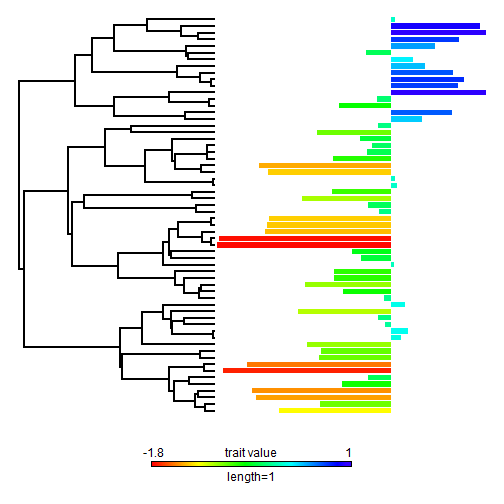Something like that….

## Friday, October 23, 2015

### Node, edge, & tip labels for plotted cophylo objects

A recent blog comment asked if there was some way to 'maintain support values' (i.e., include node, edge, or tip labels) for a plotted `"cophylo"` object produced by phytools.

This is not quite as straightforward as it might seem because, unlike for a single plotted tree, there are two sets of node, two sets of tips, two sets of edges etc.

My solution was as follows:

First, I have the internally used tree plotting function, `phylogram`, create an environmental variable `"last_plot.phylo"`, as do other tree plotting methods such as `plot.phylo` and `plotSimmap`.

Next, I had the S3 `plot` method for objects of class `"cophylo"` pull each of these two `"last_plot.phylo"` objects from their environment (one for each tree plotted) and create a new environmental variable, `"last_plot.cophylo"`, in the same environment, consisting of a simple list of these two objects.

Finally, I created a series of wrappers around `nodelabels`, `tiplabels`, and `edgelabels`, which take as input which tree is to have labels added to it, and then sets the corresponding element of `"last_plot.cophylo"` to `"last_plot.phylo"` so that `nodelabels` etc. can be called internally.

Here's a demo using the following two trees which have matching tree labels. (Remember, we can use non-matching labels too if we are prepared to supply an association matrix.)

``````library(phytools)
packageVersion("phytools")
``````
``````##  '0.5.4'
``````
``````par(mfrow=c(1,2))
plotTree(t1)
plotTree(t2)
``````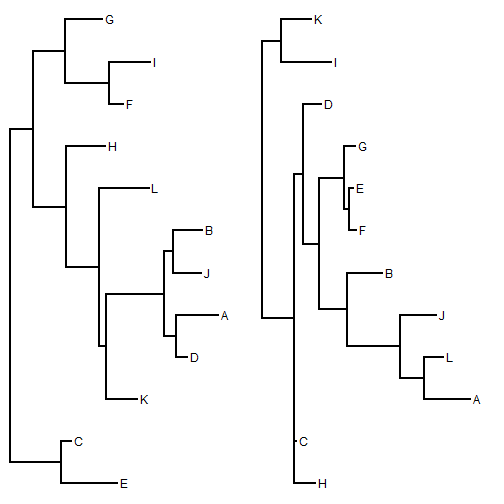Now, let's create an object of class `"cophylo"` and then try each of our new labeling methods:

``````obj<-cophylo(t1,t2)
``````
``````## Rotating nodes to optimize matching...
## Done.
``````
``````plot(obj)
nodelabels.cophylo() ## left side
nodelabels.cophylo(which="right")
``````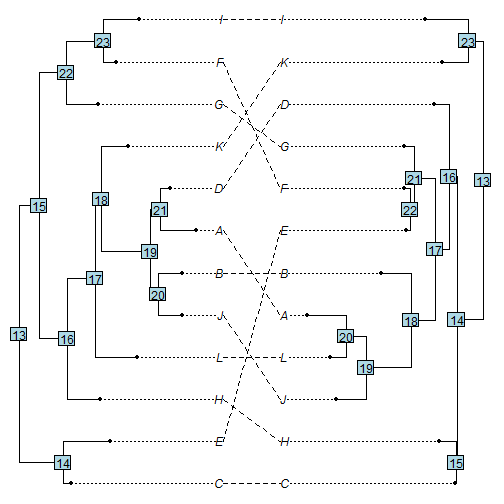We can also change the values represented, of course. For instance:

``````library(stringr)
n.left<-vector()
for(i in 1:obj\$trees[]\$Nnode){
ii<-getDescendants(obj\$trees[],i+Ntip(obj\$trees[]))
n.left[i]<-str_to_lower(paste(sort(obj\$trees[]\$tip.label[ii[ii<=Ntip(obj\$trees[])]]),
collapse=""))
}
n.right<-vector()
for(i in 1:obj\$trees[]\$Nnode){
ii<-getDescendants(obj\$trees[],i+Ntip(obj\$trees[]))
n.right[i]<-str_to_lower(paste(sort(obj\$trees[]\$tip.label[ii[ii<=Ntip(obj\$trees[])]]),
collapse=""))
}
plot(obj)
nodelabels.cophylo(n.left,cex=0.9,bg="white",srt=-90)
nodelabels.cophylo(n.right,which="right",cex=0.9,bg="white",srt=-90)
``````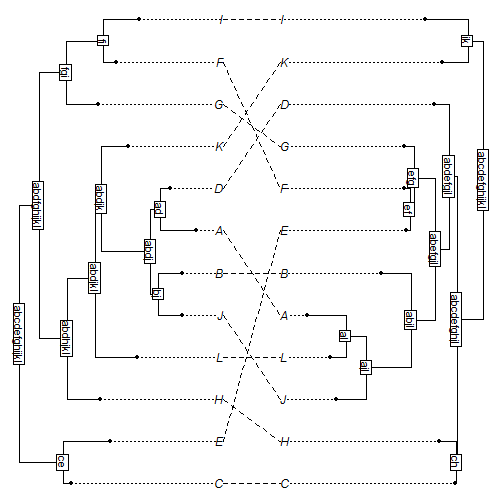Functions to label edges & tips also work for this object class, for instance:

``````plot(obj)
## show rounded edge lengths:
edgelabels.cophylo(round(obj\$trees[]\$edge.length,2),cex=0.7,
edgelabels.cophylo(round(obj\$trees[]\$edge.length,2),cex=0.7,
``````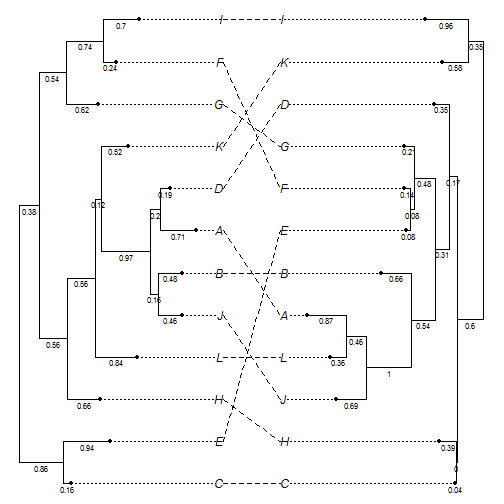``````plot(obj,ftype="off")
tiplabels.cophylo(pch=21,frame="none",bg="grey",cex=1.5)
tiplabels.cophylo(pch=21,frame="none",bg="grey",which="right",cex=1.5)
``````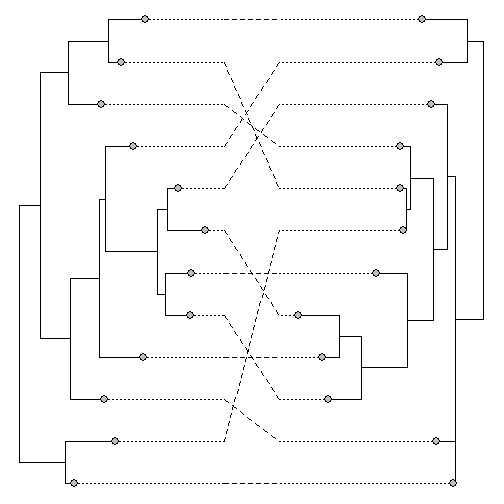We can use any of the options available from `nodelabels` and its sister functions. So, for instance, if we want to overlay reconstructed ancestral states for a discrete character, we can do so easily:

``````## tip data
x
``````
``````##   C   E   H   L   J   B   A   D   K   G   F   I
## "b" "a" "b" "b" "b" "b" "b" "a" "a" "a" "b" "a"
``````
``````y
``````
``````##   H   C   A   L   J   B   F   E   G   D   I   K
## "a" "a" "b" "b" "a" "b" "a" "a" "a" "b" "b" "b"
``````
``````fit.x<-ace(x,obj\$trees[],type="discrete")
fit.y<-ace(y,obj\$trees[],type="discrete")
## plot pies
plot(obj)
nodelabels.cophylo(pie=fit.x\$lik.anc,piecol=c("grey","white"),cex=0.7)
nodelabels.cophylo(pie=fit.y\$lik.anc,piecol=c("grey","white"),cex=0.7,
which="right")
tiplabels.cophylo(pie=to.matrix(x[obj\$trees[]\$tip.label],c("a","b")),
piecol=c("grey","white"),cex=0.4)
tiplabels.cophylo(pie=to.matrix(y[obj\$trees[]\$tip.label],c("a","b")),
piecol=c("grey","white"),cex=0.4,which="right")
``````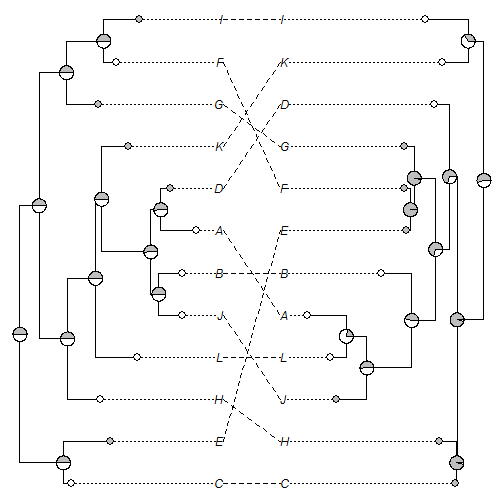Something very important to keep in mind is that the order of the tips, nodes, & edges in the object of class `"cophylo"` may be different than it was in the input trees, particulary if we set `rotate=TRUE` when we made the object.

That's it!